# Python3《机器学习实战》学习笔记（六）：Logistic回归基础篇之梯度上升算法

**机器学习知乎专栏：**https://zhuanlan.zhihu.com/ml-jack
**CSDN博客专栏：**http://blog.csdn.net/column/details/16415.html
**Github代码获取：**https://github.com/Jack-Cherish/Machine-Learning/
Python版本： Python3.x

IDE： Sublime text3

# 二 Logistic回归与梯度上升算法

Logistic回归是众多分类算法中的一员。通常，Logistic回归用于二分类问题，例如预测明天是否会下雨。当然它也可以用于多分类问题，不过为了简单起见，本文暂先讨论二分类问题。首先，让我们来了解一下，什么是Logistic回归。

## 1 Logistic回归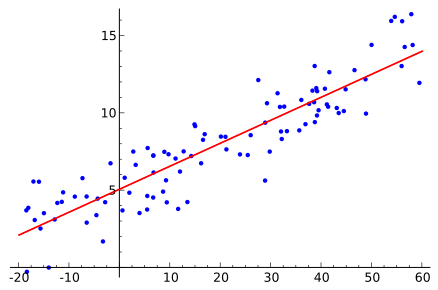Logistic回归一种二分类算法，它利用的是Sigmoid函数阈值在[0,1]这个特性。Logistic回归进行分类的主要思想是：根据现有数据对分类边界线建立回归公式，以此进行分类。其实，Logistic本质上是一个基于条件概率的判别模型(Discriminative Model)。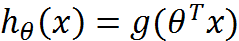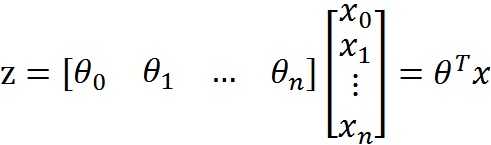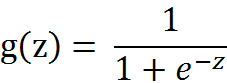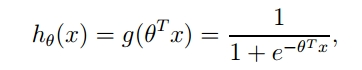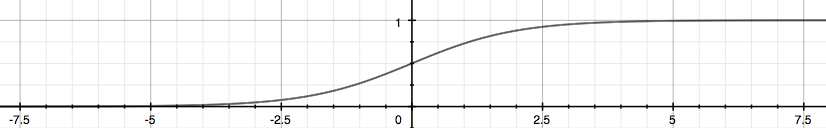z是一个矩阵，θ是参数列向量(要求解的)，x是样本列向量(给定的数据集)。θ^T表示θ的转置。g(z)函数实现了任意实数到[0,1]的映射，这样我们的数据集([x0,x1,…,xn])，不管是大于1或者小于0，都可以映射到[0,1]区间进行分类。hθ(x)给出了输出为1的概率。比如当hθ(x)=0.7，那么说明有70%的概率输出为1。输出为0的概率是输出为1的补集，也就是30%。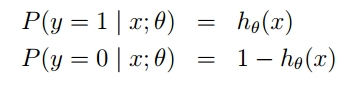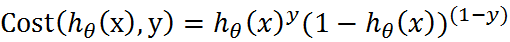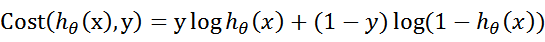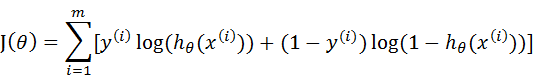## 2 梯度上升算法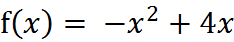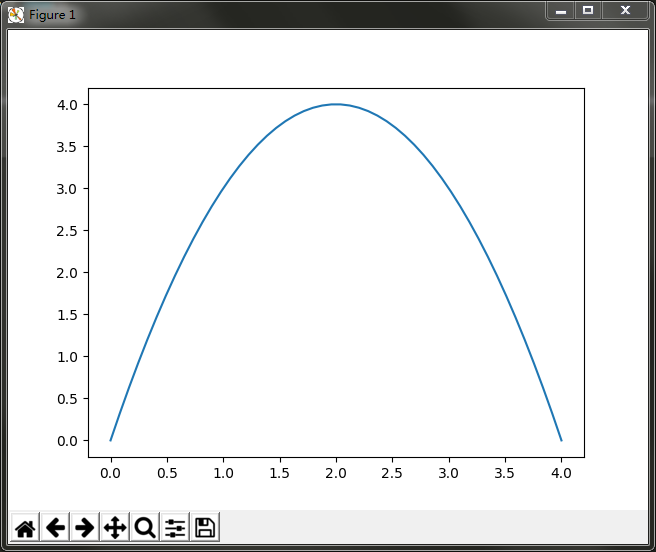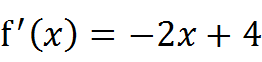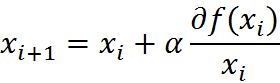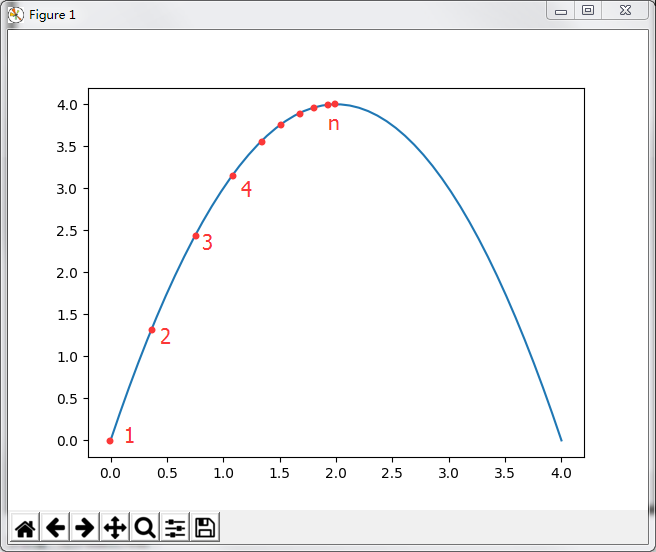# -*- coding:UTF-8 -*-
"""

Parameters:
无
Returns:
无
Author:
Jack Cui
Blog:
http://blog.csdn.net/c406495762
Zhihu:
https://www.zhihu.com/people/Jack--Cui/
Modify:
2017-08-28
"""
def Gradient_Ascent_test():
def f_prime(x_old):                                    #f(x)的导数
return -2 * x_old + 4
x_old = -1                                            #初始值，给一个小于x_new的值
x_new = 0                                            #梯度上升算法初始值，即从(0,0)开始
alpha = 0.01                                        #步长，也就是学习速率，控制更新的幅度
presision = 0.00000001                                #精度，也就是更新阈值
while abs(x_new - x_old) > presision:
x_old = x_new
x_new = x_old + alpha * f_prime(x_old)            #上面提到的公式
print(x_new)                                        #打印最终求解的极值近似值

if __name__ == '__main__':
Gradient_Ascent_test()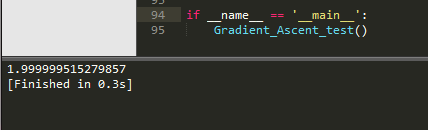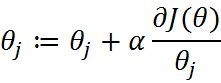sigmoid函数为：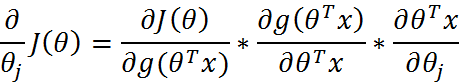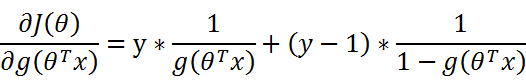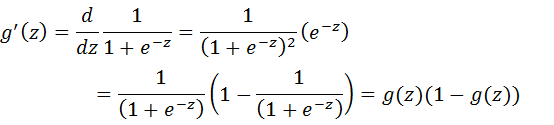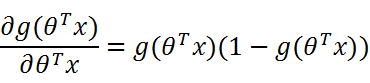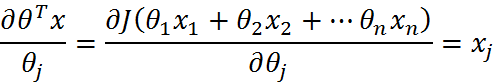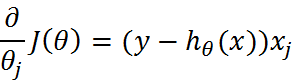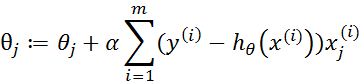# 三 Python3实战

## 1 数据准备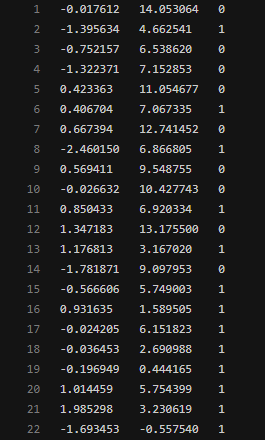# -*- coding:UTF-8 -*-
import matplotlib.pyplot as plt
import numpy as np

"""

Parameters:
无
Returns:
dataMat - 数据列表
labelMat - 标签列表
Author:
Jack Cui
Blog:
http://blog.csdn.net/c406495762
Zhihu:
https://www.zhihu.com/people/Jack--Cui/
Modify:
2017-08-28
"""
def loadDataSet():
dataMat = []                                                        #创建数据列表
labelMat = []                                                        #创建标签列表
fr = open('testSet.txt')                                            #打开文件
for line in fr.readlines():                                            #逐行读取
lineArr = line.strip().split()                                    #去回车，放入列表
dataMat.append([1.0, float(lineArr), float(lineArr)])        #添加数据
labelMat.append(int(lineArr))                                #添加标签
fr.close()                                                            #关闭文件
return dataMat, labelMat                                            #返回

"""

Parameters:
无
Returns:
无
Author:
Jack Cui
Blog:
http://blog.csdn.net/c406495762
Zhihu:
https://www.zhihu.com/people/Jack--Cui/
Modify:
2017-08-28
"""
def plotDataSet():
dataMat, labelMat = loadDataSet()                                    #加载数据集
dataArr = np.array(dataMat)                                            #转换成numpy的array数组
n = np.shape(dataMat)                                            #数据个数
xcord1 = []; ycord1 = []                                            #正样本
xcord2 = []; ycord2 = []                                            #负样本
for i in range(n):                                                    #根据数据集标签进行分类
if int(labelMat[i]) == 1:
xcord1.append(dataArr[i,1]); ycord1.append(dataArr[i,2])    #1为正样本
else:
xcord2.append(dataArr[i,1]); ycord2.append(dataArr[i,2])    #0为负样本
fig = plt.figure()
ax = fig.add_subplot(111)                                            #添加subplot
ax.scatter(xcord1, ycord1, s = 20, c = 'red', marker = 's',alpha=.5)#绘制正样本
ax.scatter(xcord2, ycord2, s = 20, c = 'green',alpha=.5)            #绘制负样本
plt.title('DataSet')                                                #绘制title
plt.xlabel('x'); plt.ylabel('y')                                    #绘制label
plt.show()                                                            #显示

if __name__ == '__main__':
plotDataSet()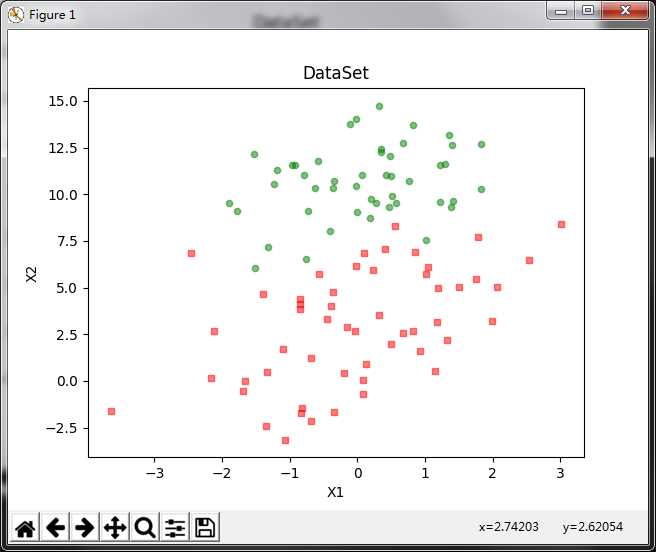## 2 训练算法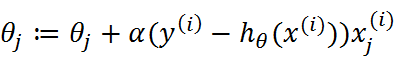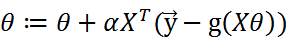# -*- coding:UTF-8 -*-
import numpy as np

"""

Parameters:
无
Returns:
dataMat - 数据列表
labelMat - 标签列表
Author:
Jack Cui
Blog:
http://blog.csdn.net/c406495762
Zhihu:
https://www.zhihu.com/people/Jack--Cui/
Modify:
2017-08-28
"""
def loadDataSet():
dataMat = []                                                        #创建数据列表
labelMat = []                                                        #创建标签列表
fr = open('testSet.txt')                                            #打开文件
for line in fr.readlines():                                            #逐行读取
lineArr = line.strip().split()                                    #去回车，放入列表
dataMat.append([1.0, float(lineArr), float(lineArr)])        #添加数据
labelMat.append(int(lineArr))                                #添加标签
fr.close()                                                            #关闭文件
return dataMat, labelMat                                            #返回

"""

Parameters:
inX - 数据
Returns:
sigmoid函数
Author:
Jack Cui
Blog:
http://blog.csdn.net/c406495762
Zhihu:
https://www.zhihu.com/people/Jack--Cui/
Modify:
2017-08-28
"""
def sigmoid(inX):
return 1.0 / (1 + np.exp(-inX))

"""

Parameters:
dataMatIn - 数据集
classLabels - 数据标签
Returns:
weights.getA() - 求得的权重数组(最优参数)
Author:
Jack Cui
Blog:
http://blog.csdn.net/c406495762
Zhihu:
https://www.zhihu.com/people/Jack--Cui/
Modify:
2017-08-28
"""
def gradAscent(dataMatIn, classLabels):
dataMatrix = np.mat(dataMatIn)                                        #转换成numpy的mat
labelMat = np.mat(classLabels).transpose()                            #转换成numpy的mat,并进行转置
m, n = np.shape(dataMatrix)                                            #返回dataMatrix的大小。m为行数,n为列数。
alpha = 0.001                                                        #移动步长,也就是学习速率,控制更新的幅度。
maxCycles = 500                                                        #最大迭代次数
weights = np.ones((n,1))
for k in range(maxCycles):
h = sigmoid(dataMatrix * weights)                                #梯度上升矢量化公式
error = labelMat - h
weights = weights + alpha * dataMatrix.transpose() * error
return weights.getA()                                                #将矩阵转换为数组，返回权重数组

if __name__ == '__main__':
dataMat, labelMat = loadDataSet()
print(gradAscent(dataMat, labelMat))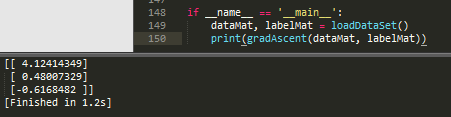## 3 绘制决策边界

# -*- coding:UTF-8 -*-
import matplotlib.pyplot as plt
import numpy as np

"""

Parameters:
无
Returns:
dataMat - 数据列表
labelMat - 标签列表
Author:
Jack Cui
Blog:
http://blog.csdn.net/c406495762
Zhihu:
https://www.zhihu.com/people/Jack--Cui/
Modify:
2017-08-28
"""
def loadDataSet():
dataMat = []                                                        #创建数据列表
labelMat = []                                                        #创建标签列表
fr = open('testSet.txt')                                            #打开文件
for line in fr.readlines():                                            #逐行读取
lineArr = line.strip().split()                                    #去回车，放入列表
dataMat.append([1.0, float(lineArr), float(lineArr)])        #添加数据
labelMat.append(int(lineArr))                                #添加标签
fr.close()                                                            #关闭文件
return dataMat, labelMat                                            #返回

"""

Parameters:
inX - 数据
Returns:
sigmoid函数
Author:
Jack Cui
Blog:
http://blog.csdn.net/c406495762
Zhihu:
https://www.zhihu.com/people/Jack--Cui/
Modify:
2017-08-28
"""
def sigmoid(inX):
return 1.0 / (1 + np.exp(-inX))

"""

Parameters:
dataMatIn - 数据集
classLabels - 数据标签
Returns:
weights.getA() - 求得的权重数组(最优参数)
Author:
Jack Cui
Blog:
http://blog.csdn.net/c406495762
Zhihu:
https://www.zhihu.com/people/Jack--Cui/
Modify:
2017-08-28
"""
def gradAscent(dataMatIn, classLabels):
dataMatrix = np.mat(dataMatIn)                                        #转换成numpy的mat
labelMat = np.mat(classLabels).transpose()                            #转换成numpy的mat,并进行转置
m, n = np.shape(dataMatrix)                                            #返回dataMatrix的大小。m为行数,n为列数。
alpha = 0.001                                                        #移动步长,也就是学习速率,控制更新的幅度。
maxCycles = 500                                                        #最大迭代次数
weights = np.ones((n,1))
for k in range(maxCycles):
h = sigmoid(dataMatrix * weights)                                #梯度上升矢量化公式
error = labelMat - h
weights = weights + alpha * dataMatrix.transpose() * error
return weights.getA()                                                #将矩阵转换为数组，返回权重数组

"""

Parameters:
weights - 权重参数数组
Returns:
无
Author:
Jack Cui
Blog:
http://blog.csdn.net/c406495762
Zhihu:
https://www.zhihu.com/people/Jack--Cui/
Modify:
2017-08-30
"""
def plotBestFit(weights):
dataMat, labelMat = loadDataSet()                                    #加载数据集
dataArr = np.array(dataMat)                                            #转换成numpy的array数组
n = np.shape(dataMat)                                            #数据个数
xcord1 = []; ycord1 = []                                            #正样本
xcord2 = []; ycord2 = []                                            #负样本
for i in range(n):                                                    #根据数据集标签进行分类
if int(labelMat[i]) == 1:
xcord1.append(dataArr[i,1]); ycord1.append(dataArr[i,2])    #1为正样本
else:
xcord2.append(dataArr[i,1]); ycord2.append(dataArr[i,2])    #0为负样本
fig = plt.figure()
ax = fig.add_subplot(111)                                            #添加subplot
ax.scatter(xcord1, ycord1, s = 20, c = 'red', marker = 's',alpha=.5)#绘制正样本
ax.scatter(xcord2, ycord2, s = 20, c = 'green',alpha=.5)            #绘制负样本
x = np.arange(-3.0, 3.0, 0.1)
y = (-weights - weights * x) / weights
ax.plot(x, y)
plt.title('BestFit')                                                #绘制title
plt.xlabel('X1'); plt.ylabel('X2')                                    #绘制label
plt.show()

if __name__ == '__main__':
dataMat, labelMat = loadDataSet()
weights = gradAscent(dataMat, labelMat)
plotBestFit(weights)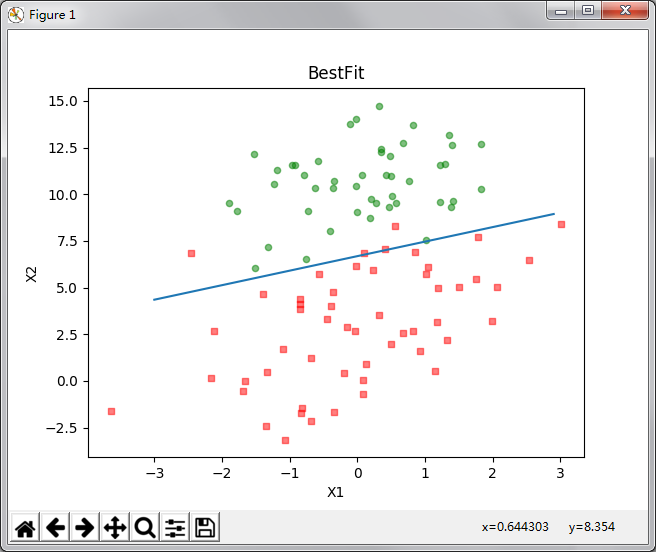# 四 总结

Logistic回归的一般过程：

• 收集数据：采用任意方法收集数据。
• 准备数据：由于需要进行距离计算，因此要求数据类型为数值型。另外，结构化数据格式则最佳。
• 分析数据：采用任意方法对数据进行分析。
• 训练算法：大部分时间将用于训练，训练的目的是为了找到最佳的分类回归系数。
• 测试算法：一旦训练步骤完成，分类将会很快。
• 使用算法：首先，我们需要输入一些数据，并将其转换成对应的结构化数值；接着，基于训练好的回归系数，就可以对这些数值进行简单的回归计算，判定它们属于哪个类别；在这之后，我们就可以在输出的类别上做一些其他分析工作。

• Logistic回归的目的是寻找一个非线性函数Sigmoid的最佳拟合参数，求解过程可以由最优化算法完成。
• 本文讲述了Logistic回归原理以及数学推导过程。
• 下篇文章将讲解Logistic回归的改进以及Sklearn实战内容。
• 如有问题，请留言。如有错误，还望指正，谢谢！

PS： 如果觉得本篇本章对您有所帮助，欢迎关注、评论、顶！